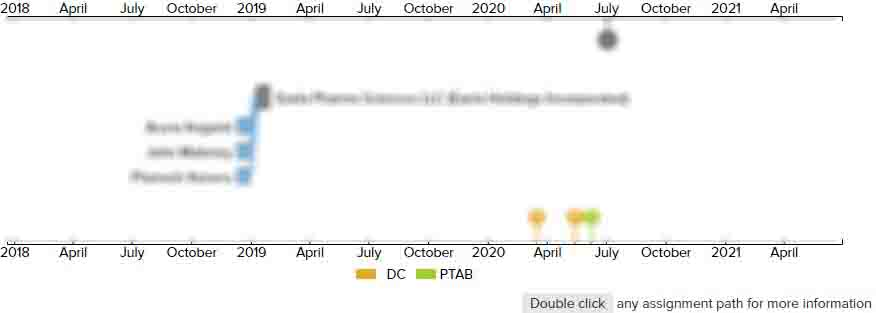# Color correction circuit, driving device, and display device

• US 20080007565A1
• Filed: 07/02/2007
• Published: 01/10/2008
• Est. Priority Date: 07/03/2006
• Status: Abandoned Application
##### First Claim
Patent Images

1. A color correction circuit for adjusting a color tone on a display device for performing color display based on a luminance of each colors R, G, and B, comprising:

• a pre-gamma circuit for converting input numerical values of the R, G, and B into linear data;

a matrix operation circuit for performing an operation using numerical values from the pre-gamma circuit; and

a post-gamma circuit for converting numerical values from the matrix operation circuit into nonlinear data, wherein a number of bits of the numerical values from the pre-gamma circuit is made larger than a number of bits of the input numerical values of each of the R, G, and B to increase a resolution.

• ##### 1 Assignment
Timeline View
Assignment View×
×